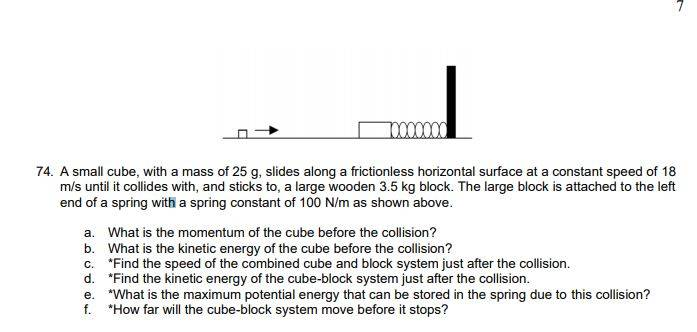# Physics momentum problem -- Collision between 2 blocks that stick together

Inwoodspaki
Homework Statement:
A small cube, with a mass of 25 g, slides along a frictionless horizontal surface at a constant speed of 18 m/s until it collides with, and sticks to, a large wooden 3.5 kg block. The large block is attached to the left end of a spring with a spring constant of 100 N/m as shown above.

a. What is the momentum of the cube before the collision?
b. What is the kinetic energy of the cube before the collision?
c. *Find the speed of the combined cube and block system just after the collision.
d. *Find the kinetic energy of the cube-block system just after the collision.
e. *What is the maximum potential energy that can be stored in the spring due to this collision?
f. *How far will the cube-block system move before it stops?
Relevant Equations:
KE = 1/2 mv^2
P= mv

maybe D = (KE) / (m)(g) ?
So far I found the answer for a and b, but when I attempted to do the other ones I was completely lost.A.) P= MV
M = 25g = .025kg
V = 18

.025 * 18 = .45kg*m/s

B.) KE= 1/2 mv^2
1/2 (.025)(18)^2
4.05 J

Homework Helper
Gold Member
2022 Award
For c) what general principle of mechanics might be relevant? Hint: you're looking for something that is conserved throughout the collision.